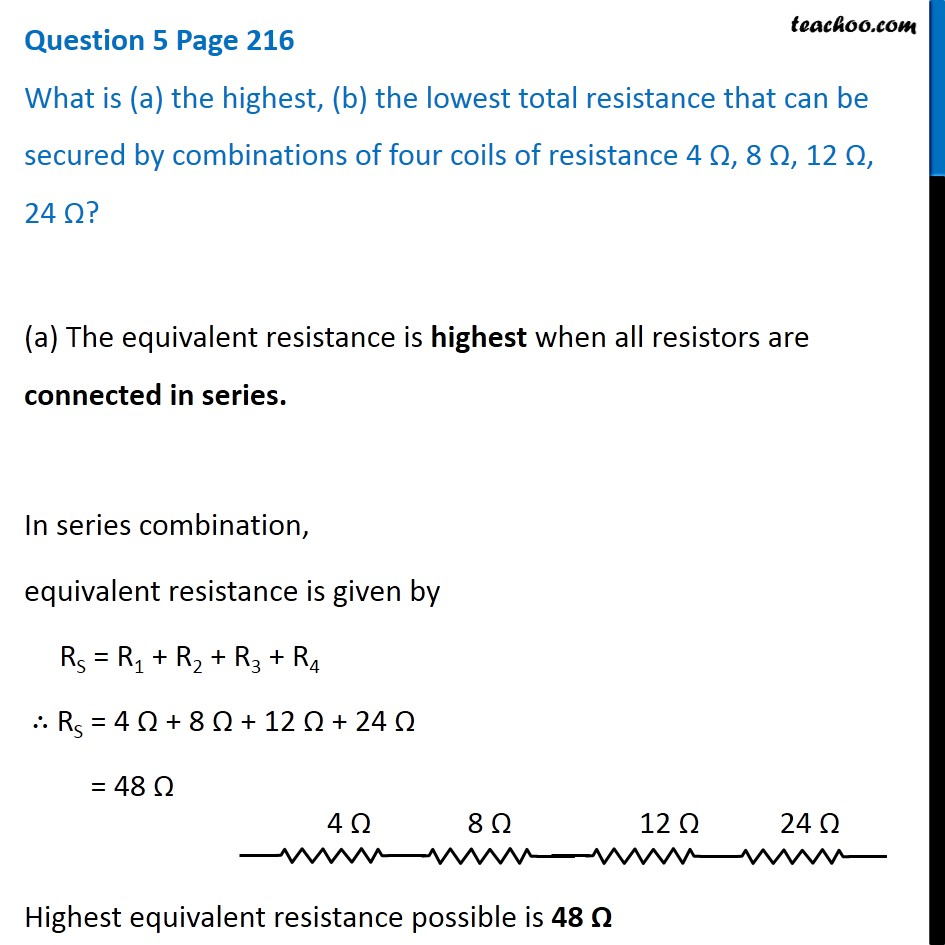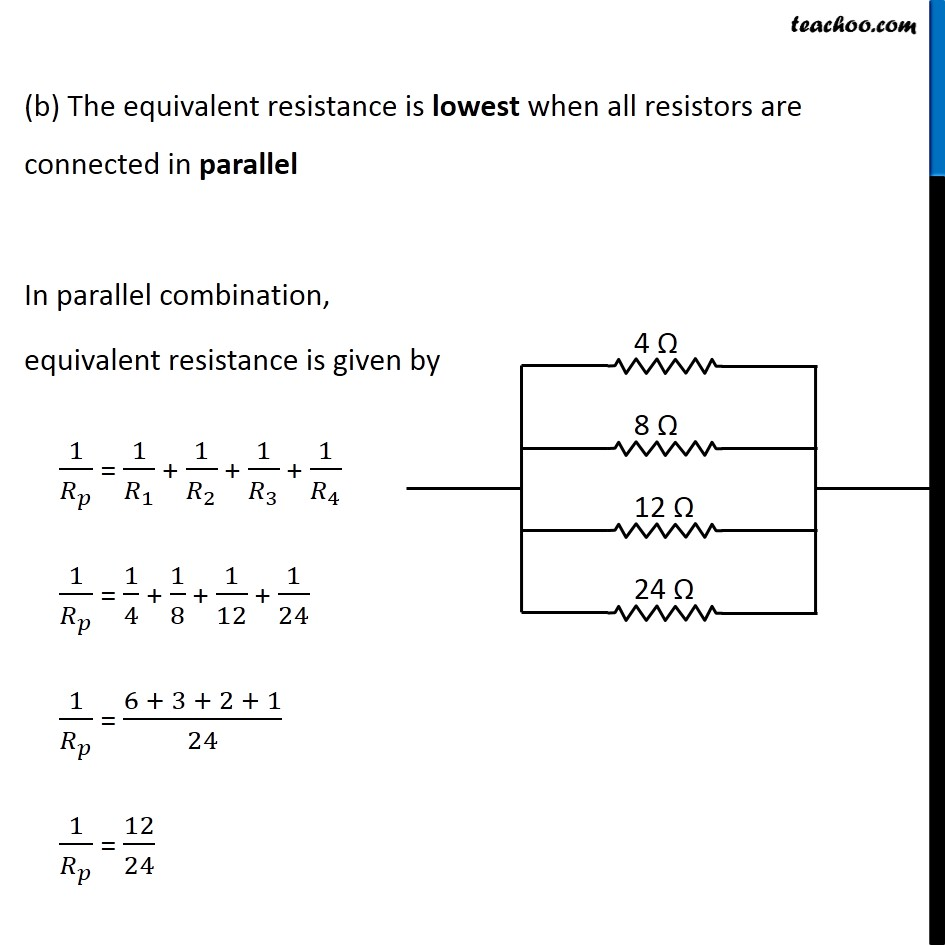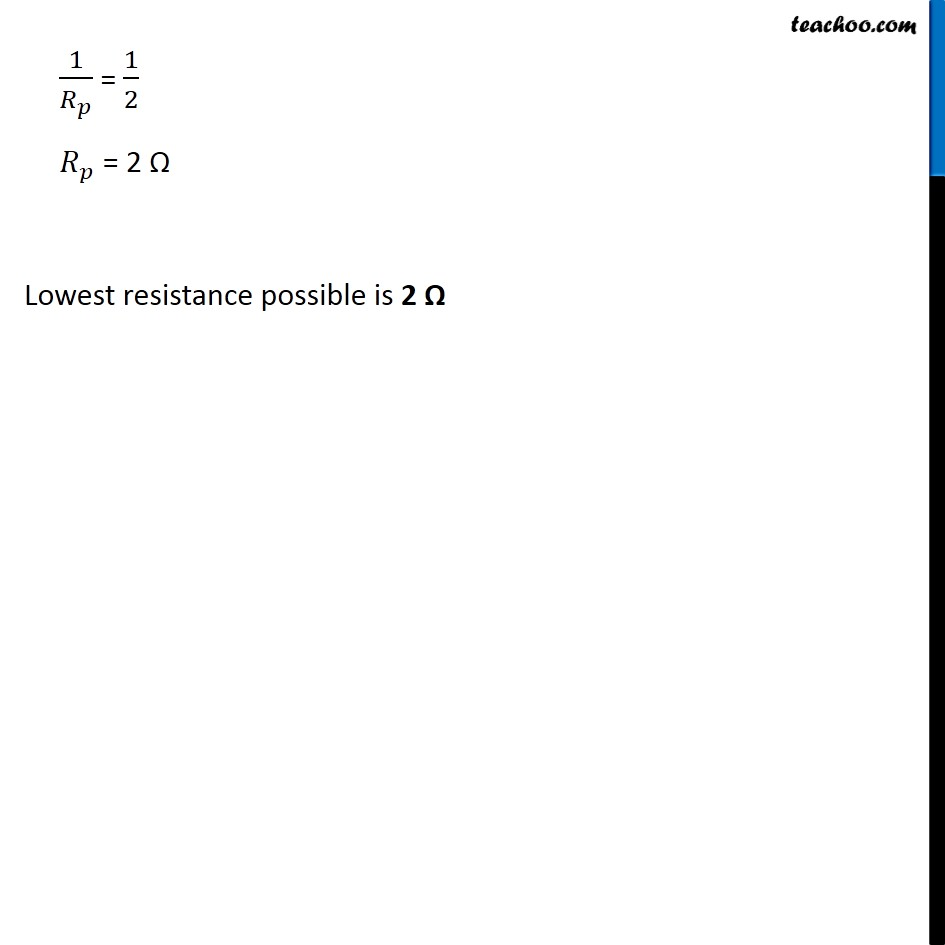Questions from Inside the chapter

Class 10
Chapter 12 Class 10 - Electricity

## What is (a) the highest, (b) the lowest total resistance that can be secured by combinations of four coils of resistance 4 Ω, 8 Ω, 12 Ω, 24 Ω?Learn in your speed, with individual attention - Teachoo Maths 1-on-1 Class

### Transcript

Question 5 Page 216 What is (a) the highest, (b) the lowest total resistance that can be secured by combinations of four coils of resistance 4 Ω, 8 Ω, 12 Ω, 24 Ω? (a) The equivalent resistance is highest when all resistors are connected in series. In series combination, equivalent resistance is given by RS = R1 + R2 + R3 + R4 ∴ RS = 4 Ω + 8 Ω + 12 Ω + 24 Ω = 48 Ω Highest equivalent resistance possible is 48 Ω (b) The equivalent resistance is lowest when all resistors are connected in parallel In parallel combination, equivalent resistance is given by 1/𝑅_𝑝 = 1/𝑅_1 + 1/𝑅_2 + 1/𝑅_3 + 1/𝑅_4 1/𝑅_𝑝 = 1/4 + 1/8 + 1/12 + 1/24 1/𝑅_𝑝 = (6 + 3 + 2 + 1)/24 1/𝑅_𝑝 = 12/24 1/𝑅_𝑝 = 1/2 𝑅_𝑝 = 2 Ω Lowest resistance possible is 2 Ω